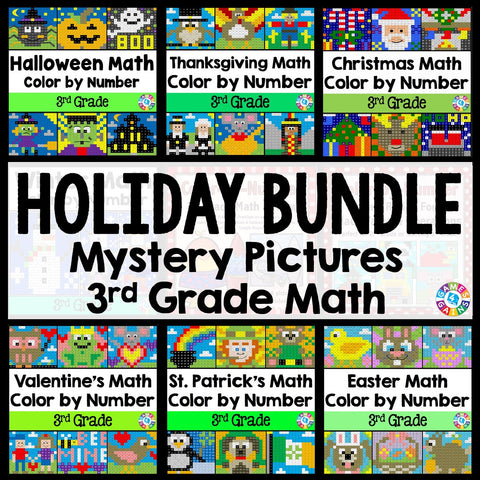## 3rd Grade Math Color-by-Number Holiday Bundle

• \$3400
• Save \$8.45

This 3rd Grade Math Color-by-Number Holiday Bundle comes to you after receiving numerous requests from buyers to create a bundle that includes all of my seasonal 3rd grade math color-by-number sets!

This HUGE bundle covers a wide variety of 3rd grade math skills and includes 52 mystery pictures for celebrating all of the major holidays during the school year. Students will have a blast each holiday as they practice key math skills in a fun and COLORFUL way!

Plus, you will SAVE \$\$\$ when you purchase this bundle. If you bought each holiday set individually, you would spend \$40.

Want to learn more about what's included in this bundle? Click on the links below to view the individual previews for each activity. Remember, you'll get all of these holiday sets when you purchase this bundle!

"School Days" Place Value and Operations Color-by-Number Activities (2nd/3rd Grade)

*Note: This set is a REVIEW of 2nd grade math skills, so that 3rd grade teachers can use these to review with their incoming 3rd graders.

• Skip-counting by 5s, 10s, and 100s (within 1,000)
• Identifying the place value of three-digit numbers
• Writing three-digit numbers in different forms
• Adding 10 or 100 to a given number 100-900 and subtracting 10 or 100 from a given number 100-900
• Adding within 1,000
• Subtracting within 1,000

Halloween Math Color-by-Number Activities (3rd Grade)

• Writing multiplication equations for arrays
• Determining the missing number while skip counting
• Solving one-step multiplication word problems (facts for 2-5, 10 only)
• Rounding numbers to the nearest ten and hundred
• Adding numbers within 1,000
• Subtracting numbers within 1,000

Thanksgiving Math Color-by-Number Activities (3rd Grade)

• Estimating sums and differences
• Subtracting across zeros
• Solving two-step addition and subtraction word problems
• Multiplying in different forms (groups, repeated addition, etc.)
• Solving multiplication facts
• Finding the unknown in division facts

Christmas Math Color By Number (3rd Grade)

• Multiplying basic facts
• Dividing basic facts
• Solving one-step multiplication and division word problems
• Writing basic fractions (parts of a set)
• Telling time to the nearest minute
• Interpreting tally charts, picture graphs, and bar graphs

Winter Math Color By Number (3rd Grade)

• Rounding numbers to 10s and 100s word problems
• Adding numbers within 1,000 word problems
• Subtracting numbers within 1,000 word problems
• Multiplying by multiples of 10 word problems

Valentine's Day Math Color-by-Number Activities (3rd Grade)

• Determining the missing number in addition and subtraction problems within 1,000
• Determining the missing number in multiplication and division facts
• Naming the fraction from a picture
• Naming the fraction from a number line
• Solving problems using a bar graph and picture graph
• Determining how many minutes have elapsed when given a start time and end time (within 1 hour)

St. Patrick's Day Math Color-by-Number Activities (3rd Grade)

• Solving multi-step word problems with all operations
• Determining equivalent fractions
• Determining the start time and end time
• Estimating metric measurements of capacity and weight (mL, L, g, kg)
• Measuring to the nearest inch, half inch, and quarter inch
• Identifying polygons based on their characteristics

Easter Math Color-by-Number Activities (3rd Grade)

• Determining the unknown in multiplication by multiples of 10 equations
• Determining the next number in an addition or subtraction pattern
• Comparing fractions with the same denominator or same numerator
• Solving word problems for metric mass and capacity (g, kg, mL, L)
• Calculating the perimeter and area of rectangles on a grid
• Solving problems using line plots (with units by 1/4 and 1/2)

Summer Math Color-by-Number Activities (3rd Grade)

• Writing equivalent fractions and identifying fractions on a number line
• Finding the unknown in basic multiplication and division facts
• Adding and subtracting within 1,000
• Determining the duration, start time, and end time in elapsed time problems
• Calculating the perimeter and area
• Measuring lengths to the nearest half and quarter inch